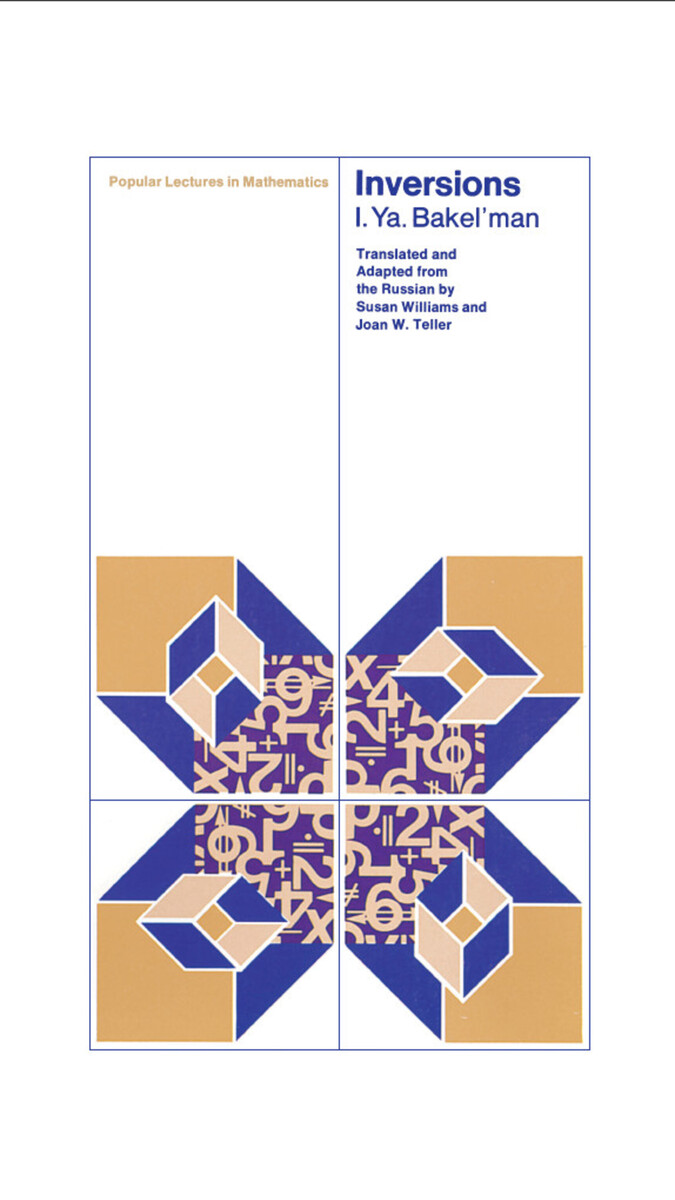Inversions
by I. Ya Bakel'man
translated by Joan W. Teller and Susan Williams
University of Chicago Press, 1975
Paper: 978-0-226-03499-7
Library of Congress Classification QA473.B3413
Dewey Decimal Classification 516.1

ABOUT THIS BOOK | REQUEST ACCESSIBLE FILE
ABOUT THIS BOOK
In this book, I. Ya. Bakel'man introduces inversion transformations in the Euclidean plane and discusses the interrelationships among more general mathematical concepts. The author begins by defining and giving examples of the concept of a transformation in the Euclidean plane, and then explains the "point of infinity" and the "stereographic projection" of the sphere onto the plane. With this preparation, the student is capable of applying the theory of inversions to classical construction problems in the plane.

The author also discusses the theory of pencils of circles, and he uses the acquired techniques in a proof of Ptolemy's theorem. In the final chapter, the idea of a group is introduced with applications of group theory to geometry. The author demonstrates the group-theoretic basis for the distinction between Euclidean and Lobachevskian geometry.

See other books on: Geometry | Mathematics
See other titles from University of Chicago Press
Nearby on shelf for Mathematics / Geometry. Trigonometry. Topology: